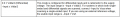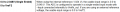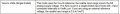#### ElectricSpidey

Joined Dec 2, 2017
2,639
So I'm trying to get an ADC SAR that is embedded in the PSoC 5lp to work, but I'm not getting the results I expect, so in an effort to narrow it down to my code or my assumptions about the ADC, I would like the following questions answered.

Resolution is 8bit
Input range is 0 to 5 volts
Reference voltage is 2.5

The data sheets states...
The output is the input minus the reference, and is in 2s compliment.

So, can I assume any voltage input from 0 to 2.5 will will output a value from 0 to -127?
Any input voltage from 2.5 to 5 will output a positive value from 0 to 127?

And if I want a positive value output from 0 to 255 I need to make the reference voltage "0"?

Are these assumptions correct, if not please explain.

#### Irving

Joined Jan 30, 2016
3,548
So I'm trying to get an ADC SAR that is embedded in the PSoC 5lp to work, but I'm not getting the results I expect, so in an effort to narrow it down to my code or my assumptions about the ADC, I would like the following questions answered.

Resolution is 8bit
Input range is 0 to 5 volts
Reference voltage is 2.5

The data sheets states...
The output is the input minus the reference, and is in 2s compliment.

So, can I assume any voltage input from 0 to 2.5 will will output a value from 0 to -127?
Any input voltage from 2.5 to 5 will output a positive value from 0 to 127?

And if I want a positive value output from 0 to 255 I need to make the reference voltage "0"?

Are these assumptions correct, if not please explain.
According to datasheet:

The PSoC 5LP family of devices has a SAR ADC. This ADC is 12-bit at up to 1 Msps, with single-ended or differential inputs, making it useful for a wide variety of sampling and control applications.

The bigger devices have 2 SAR ADC.

The PSoC SAR software component allows you to configure the ADC as 8, 10 or 12bit.

You can configure the SAR to be single-ended with the -ve input connected to the 0v rail, in which case the digital value output is 0 - vref * 2 where vref is one of: the internal 1.024v reference, an external voltage < VDDA/2, or VDDA/2.

#### ElectricSpidey

Joined Dec 2, 2017
2,639
Thanks, the setting I'm using is locked at 2.5 volts reference (VDDA/2)

So I got it working, and it was my assumptions about the ADC.

So instead of the first 0 to 2.5 volts outputting a negative value, it instead puts out a positive value.

So that leaves me without a clue what "The conversion result is a function of the +Input signal minus the voltage reference." means.

So, if the input signal is 1 volt and the reference is 2.5 volts the result should be negative...right?
1 minus 2.5 = 1.5-
And if the input signal is 3 volts and the reference is 2.5 the results will be positive.
3 minus 2.5 = .5

#### bogosort

Joined Sep 24, 2011
696
Thanks, the setting I'm using is locked at 2.5 volts reference (VDDA/2)
Found this:From the description, it seems that VDDA/2 mode assumes differential inputs and is optimized for input signals that swing between -VDDA/2 and +VDDA/2, centered at 0 V. What do you have the -Input tied to?

So instead of the first 0 to 2.5 volts outputting a negative value, it instead puts out a positive value.
That tells me that you're using half of the dynamic range. As you have it configured, the ADC will convert input voltages in the range [-2.5, 0) to negative codes, but I'm guessing your input never goes below 0 V, so you're only using 7 of the 8 conversion bits.

If you're using a single-ended input, why not put the ADC in single-ended mode? Then you can choose a VREF that optimizes for the dynamic range of your input signal.So, for example, if your input's full-scale range is 0 to 5 V, then setting VREF = 2.5 V will give you what you expect: input voltages in the range [0, 2.5) will produce negative (two's complement) codes in the range [0x80, 0xFF]. Inputs in the range [2.5, 5.0) will produce positive codes in the range [0x00, 0x7F].

#### ElectricSpidey

Joined Dec 2, 2017
2,639
Yes thanks, I have all of that information.

I'm using single ended mode.

No inputs can go below 0 volts the reference is a sort of virtual ground.

-input is tied internal to Vrefhi and is fixed @ 2.5 for the configuration I'm using. I don't have a stable external reference voltage that is why I'm stuck with the internal of 2.5.

I am only using half the usable range, that's all I really need, my puzzlement was this quote, and what I assumed it meant.

" The conversion result is a function of the +Input signal minus the voltage reference. "

Which I assumed would produce negative values, when the input was from 0 to 2.5 and positive when the input was from 2.5 to 5.

But, the output is positive with the input from 0 to 2.5 volts, that's where my confusion comes from.

If someone could just explain what that quote means....

#### ElectricSpidey

Joined Dec 2, 2017
2,639
Ok, so why does this code work?

Is it the compiler dropping the negative sign from the results, because it knows I'm an idiot?
Does it have to do with using decimal instead of binary? (I'm using a signed integer as the var type IE: int16)
Does it not care if decimal numbers are negative or positive?

I'm using the bottom range (0 to 2.5) and using positive numbers in my code.

I really need to understand this.

BTW I switched to 10 bit.

Code:
        SAR_2_Start();
SAR_2_StartConvert(); CyDelay(10);

while(L2 > 0)
{G2 = SAR_2_GetResult16();
if (G2 <  51)                 {P1 = 15; P2 = 20; Volume_1 }
if((G2 >= 50)  && (G2 < 100)) {P1 = 20; P2 = 25; Volume_2 }
if((G2 >= 100) && (G2 < 150)) {P1 = 25; P2 = 30; Volume_3 }
if((G2 >= 150) && (G2 < 200)) {P1 = 30; P2 = 35; Volume_4 }
if((G2 >= 200) && (G2 < 250)) {P1 = 35; P2 = 40; Volume_5 }
if((G2 >= 250) && (G2 < 300)) {P1 = 40; P2 = 45; Volume_6 }
if((G2 >= 300) && (G2 < 350)) {P1 = 45; P2 = 50; Volume_7 }
if((G2 >= 350) && (G2 < 400)) {P1 = 50; P2 = 55; Volume_8 }
if((G2 >= 400) && (G2 < 450)) {P1 = 55; P2 = 60; Volume_9 }
if (G2 >= 450)                {P1 = 60; P2 = 65; Volume_10}
--L2; CyDelay(500);}
SAR_2_Stop(); Volume_Display_OFF

#### bogosort

Joined Sep 24, 2011
696
I am only using half the usable range, that's all I really need, my puzzlement was this quote, and what I assumed it meant.

" The conversion result is a function of the +Input signal minus the voltage reference. "

Which I assumed would produce negative values, when the input was from 0 to 2.5 and positive when the input was from 2.5 to 5.

But, the output is positive with the input from 0 to 2.5 volts, that's where my confusion comes from.

If someone could just explain what that quote means....
The full quote is this: " The conversion result is a function of the +Input signal minus the voltage reference. The voltage reference is either the –Input signal or VSSA." Your assumption would be true if the reference was 2.5 V (-Input tied to VDD/2), but what you're seeing is what happens when the reference is tied to VSSA, which I believe is analog ground.

In other words, your ADC is calculating the expression +Input - 0, which is simply +Input. Since your input is never negative, you're only seeing positive output codes.

#### bogosort

Joined Sep 24, 2011
696
Ok, so why does this code work?
I don't have enough context of your code to know why it shouldn't work. What's the actual problem?

Is it the compiler dropping the negative sign from the results, because it knows I'm an idiot?
Does it have to do with using decimal instead of binary? (I'm using a signed integer as the var type IE: int16)
Does it not care if decimal numbers are negative or positive?
What negative sign? Your ADC is producing only positive output codes, right? If SAR_2_GetResult16() is just a simple wrapper around ADC_GetResult16(), then it will return a sign-extended 16-bit version of the ADC's 10-bit output, meaning it will respect the sign of the sample value. But all your samples are positive.

I'm not sure what you mean by "decimal numbers". You don't show how the variables were declared, but all the standard integer types and numeric literals are "binary".

BTW, I'd change all but the first of the if statements to "else if". Once a condition is matched, no need to waste cycles checking the other conditions. The compiler might handle this for you, but I'd be explicit.

#### ElectricSpidey

Joined Dec 2, 2017
2,639
Well according to the IDEs configuration dialog box, the reference is 2.5 volts, and is grayed out.

This is the input mode I'm using (just not all of it)Decimal numbers simply means that is how I enter them into my code...the complier converts them to binary for me...right? (or hex)

The variable "G2" is declared as a signed integer, as required by the editor. (int16)

Thanks for the tip on the code, but I'm not really worried about wasting cycles...that code only runs for a few seconds at the beginning, before the main for() loop starts, but I will keep that in mind, I'm still a total noob at coding. (and obviously at reading and understanding PSoC datasheets)

#### djsfantasi

Joined Apr 11, 2010
9,131
How do you declare G2? Is it an unsigned int? Or a signed int? Or something else?

UPDATE: Oops, I missed the definition in the previous post!

Last edited:

#### ElectricSpidey

Joined Dec 2, 2017
2,639
The variable "G2" is declared as a signed integer, as required by the editor. (int16)

#### bogosort

Joined Sep 24, 2011
696
Well according to the IDEs configuration dialog box, the reference is 2.5 volts, and is grayed out.

This is the input mode I'm using (just not all of it)

View attachment 222311
That ADC datasheet is too vague to be definitive, so I'd simply go by what I'm seeing. I'd make a simple test and see what codes I got for these input values: 0 V, 1.5 V, 2.5, 3.5 V.

If the input is being referenced to 2.5 V, then the corresponding (signed) output values should be negative, negative, 0, positive.

If the input is being referenced to analog ground, then the output values will all be non-negative.

Decimal numbers simply means that is how I enter them into my code...the complier converts them to binary for me...right? (or hex)
Ah yeah, there's a difference between the external representation of a number (like base-10, binary, hex, etc.) and how that number is internally stored, manipulated, and interpretted by the CPU. The latter is determined by the type you used in the variable's definition. You can use any representation you like that's supported by the compiler, e.g., 255, 0xFF, 0377, and 0b11111111 all translate to the same internal representation.

The important stuff is how the values are interpreted. If we assign 255 (or 0xFF, etc.) to a variable declared as a 16-bit signed integer, the CPU will interpret the value as 255. If we assign the same 255 (or 0xFF, etc.) to another variable declared as an 8-bit signed integer, the CPU will interpret the value as -1.

Thanks for the tip on the code, but I'm not really worried about wasting cycles...that code only runs for a few seconds at the beginning, before the main for() loop starts, but I will keep that in mind, I'm still a total noob at coding. (and obviously at reading and understanding PSoC datasheets)
Don't feel bad about your confusion from that ADC datasheet, it's a terrible datasheet. Look at practically any standalone ADC datasheet and you'll see that they explicitly describe every configuration option, interface behavior, and provide plenty of examples, leaving no guesswork or ambiguity in how to use the thing.